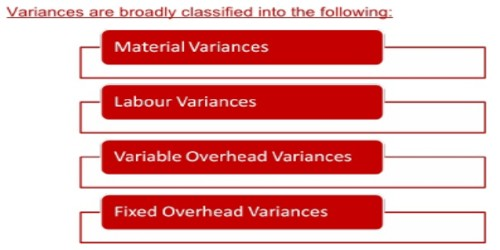Economics

# Concept of Variance Analysis and Types of VariancesConcept of Variance Analysis and Types of Variances

The terms variance refers to the deviation of the actual costs from the standard costs due to various causes. In accounting, a variance is the difference between an expected or planned amount and an actual amount. For example, a variance can occur for items contained in a department’s expense report. Variance analysis attempts to identify and explain the reasons for the difference between a budgeted amount and an actual amount.

The primary objective of variance analysis is to exercise cost control and cost reduction. Under standard costing system, the management by exception principle is applied through variance analysis. The variances are related to efficiency. Variance analysis is the process of calculating the deviation of the actual costs from the standards and of interpreting the results. Variance analysis helps to ascertain the magnitude of each of the variances and causes of variance so that corrective actions can be taken.

For three main elements of costs, variances are to be calculated and analyzed- material, labor and overheads.

Variance analysis for manufacturing overhead costs is more complicated than the variance analysis for materials. However, the variance analysis of manufacturing overhead costs is very important as manufacturing overhead costs have become a very large percentage of a product’s costs.

Types of Variances

1. Material Variance
• material cost variance
• material price variance
• material usage variance
• material mix variance
• material yield variance
1. Labor Variance
• labor cost variance
• labor rate variance
• labor efficiency variance
• labor idle time variance
• labor mix variance
• labor yield variance

A brief explanation of the above mentioned variances are presented below:

Material Cost Variance: It is the difference between actual cost of materials used and the standard cost for the actual output.

Labour Cost Variance: It is the difference between the actual direct wages paid and the direct labour cost allowed for the actual output to be achieved.

Overhead Variance: Overhead variance is the difference between the standard cost of overhead allowed for actual output (in terms of production units or labour hours) and the actual overhead cost incurred.

Controllable Variance: A variance is controllable whenever an individual or a department or section or division may be held responsible for that variance.

Information Source: